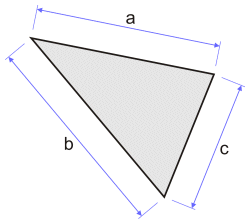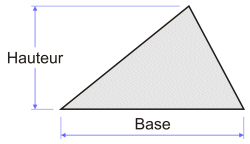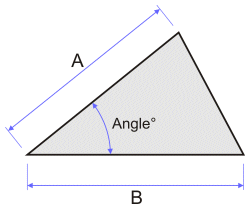# Calculating the area of triangles

Calculating the surface area of a triangle can be done using multiple methods; it all depends on the information you have available at first, thus, the module below lets you calculate this using the information at your disposal.

Three different methods:
1. Area of the triangle with the three sides (by the triangulation method)
2. Area of the triangle with the base and the height
3. Area of the triangle with two sides and their angle

## 1. area of a triangle by the triangulation method (with the length of the three sides)Length of the side a: Length of the side b: Length of the side c: Units: inch foot yard mile millimeter centimeter decimeter meter décameter hectometer kilometer Decimal places: 0 1 2 3 4 5 6 7 8 9 10 Surface of the triangle: 0,43 m2 Perimeter: 3,00 m

## 2. area of the triangle with the base and the heightLength of the base B: Height H: Units: inch foot yard mile millimeter centimeter decimeter meter décameter hectometer kilometer Decimal places: 0 1 2 3 4 5 6 7 8 9 10 Surface of the triangle: 0,50 m2

## 3. Calculating the area with two sides and their angleLength of the side A: Length of the side B: Units: inch foot yard mile millimeter centimeter decimeter meter décameter hectometer kilometer Angle between these 2 sides: Degrees Radians Gradians Decimal places: 0 1 2 3 4 5 6 7 8 9 10 Surface of the triangle: 0,35 m2